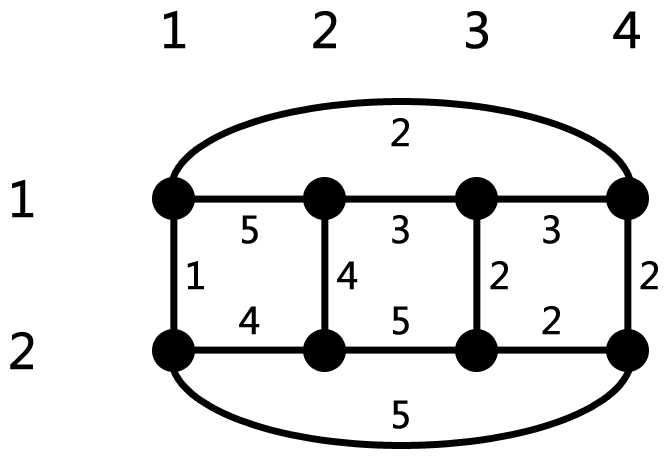시간 제한 메모리 제한 제출 정답 맞은 사람 정답 비율
4 초 512 MB 3 2 2 100.000%

## 문제

There are n × m cells on a grid, the top left cell is at (1, 1) while the bottom right cell is at (n, m).

Two cells are considered adjacent if they share a common side. Note that the grid is circular: (i, m) is also adjacent to (i, 1). However, there is no such rule for connecting the first row and the last row.

• For every cell (i, j) such that 1 ≤ i ≤ n and 1 ≤ j ≤ m, there is a road between it and (i, j mod m + 1). Note that the grid is circular, so when j = m, there is a road between (i, m) and (i, 1).
• For every cell (i, j) such that 1 ≤ i < n and 1 ≤ j ≤ m, there is a road between it and (i + 1, j).

The roads form a weighted graph, every road has its weight. You will be given q queries. In the i-th query, you will be given two integers, li and ri. Consider the graph that remains if we remove all cells (x, y) such that 1 ≤ x ≤ n and li ≤ y ≤ ri, together with the roads linked to them. You need to find the total weight of the minimum spanning tree (MST) of the resulting graph.

Note that different queries are independent. In other words, the removed cells and roads come back after you answer a query.

## 입력

There is only one test case in each test.

The first line of this test case contains six integers n, m, SA, SB, SC , and lim (1 ≤ n ≤ 100, 4 ≤ m ≤ 10 000, and 1 ≤ SA, SB, SC, lim ≤ 109).

Since the grid might be rather large, you have to generate the input using the following C/C++ code, where addedge(a, b, c, d, w) means that we add a road between (a, b) and (c, d) with weight w:

unsigned int SA, SB, SC; int lim;
int getweight() {
SA ^= SA << 16;
SA ^= SA >> 5;
SA ^= SA << 1;
unsigned int t = SA;
SA = SB;
SB = SC;
SC ^= t ^ SA;
return SC % lim + 1;
}
void gen() {
scanf("%d%d%u%u%u%d", &n, &m, &SA, &SB, &SC, &lim);
int i, j, w;
for (i = 1; i <= n; i++)
for (j = 1; j <= m; j++) {
w = getweight();
if (j < m) {
addedge(i, j, i, j + 1, w);
} else {
}
}
for (i = 1; i < n; i++)
for (j = 1; j <= m; j++) {
w = getweight();
addedge(i, j, i + 1, j, w);
}
}


A note for coders in other languages: int is a 32-bit signed integer type, whereas unsigned int is a 32-bit unsigned integer type. The result of every operation with unsigned int is truncated to the last 32 bits. This effectively means each result is taken modulo 232.

On the second line, there is one integer q (1 ≤ q ≤ 10 000), denoting the number of queries.

Each of the next q lines contains two integers li and ri (1 < li ≤ ri < m) describing a query.

## 출력

For each query, print a single line containing an integer: the total weight of the MST of the respective graph.

## 예제 입력 1

2 4 1 2 3 5
3
2 2
2 3
3 3


## 예제 출력 1

9
5
13


## 힌트The generated graph in the example.